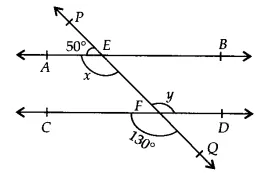Q

# In Fig. 6.28, find the values of x and y and then show that AB || CD.

1. In Fig. 6.28, find the values of x and y and then show that AB CD.ViewsGiven that,
In the figure, CD and PQ intersect at  F
Therefore,   (vertically opposite angles)

PQ is a straight line. So,

Hence AB || CD (since  and are  alternate interior angles)

Exams
Articles
Questions